# 满满干货！手把手教你实现基于eTS的分布式计算器 原创精华

HarmonyOS开发者

1收藏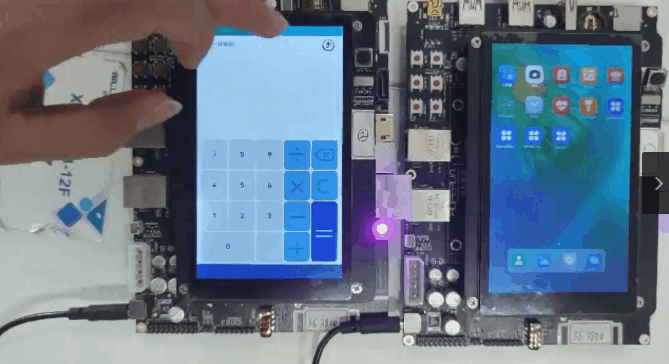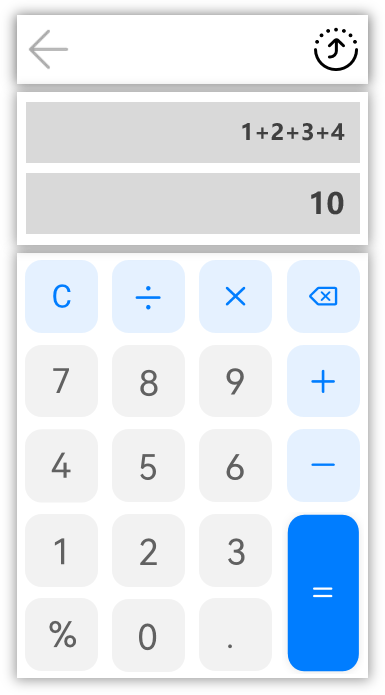1. 组件化

ArkUI开发框架定义了一些具有特殊含义的组件管理装饰器，如图2所示：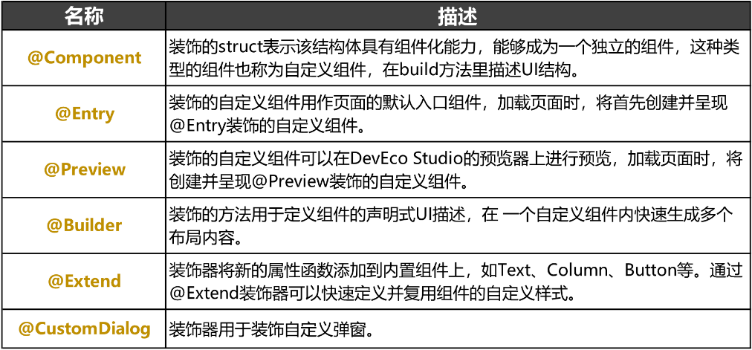2. 声明式描述

3. 状态管理

ArkUI开发框架定义了一些具有特殊含义的状态管理装饰器，如图3所示: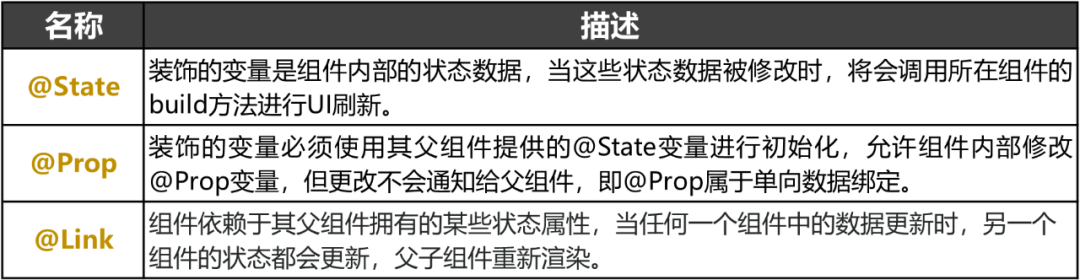1. 键盘模块

(1) 键盘布局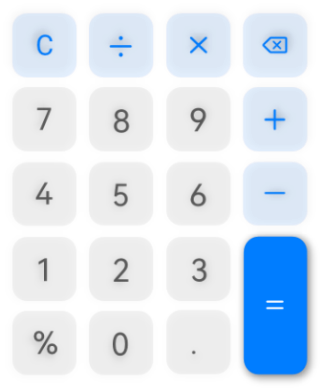``````export function obtainImgVertical(): Array<Array<ImageList>> {
let list =
[
[
{ img: \$r('app.media.ic_cal_seven'), value: '7' },
{ img: \$r('app.media.ic_cal_eight'), value: '8' },
{ img: \$r('app.media.ic_cal_nine'), value: '9' }
],
[
{ img: \$r('app.media.ic_cal_four'), value: '4' },
{ img: \$r('app.media.ic_cal_five'), value: '5' },
{ img: \$r('app.media.ic_cal_six'), value: '6' }
],
]
return list
}
export function obtainImgV(): Array<ImageList> {
let list =
[
{ img: \$r('app.media.ic_cal_delete'), value: '' },
{ img: \$r('app.media.ic_cal_minus'), value: '-' },
{ img: \$r('app.media.ic_cal_plus'), value: '+'  },
{ img: \$r('app.media.ic_cal_equal'), value: '=' }
]
return list
}``````

``````@Component
export struct ButtonComponent {
private isLand: boolean
private onInputValue: (result) => void
build() {
Row() {
Grid() {
ForEach(obtainImgV(), (item, index) => {
GridItem() {
Image(item.Img)
.margin({ top: 5 })
.onClick(() => {
this.onInputValue(item.value)
})
}
.rowStart(index)
.rowEnd(index === 3 ? index + 1 : index)
.columnStart(3)
.columnEnd(3)
})
ForEach(obtainImgVertical(), (item) => {
ForEach(item, (item) => {
GridItem() {
Image(item.Img)
.margin({ top: 5 })
.onClick(() => {
this.onInputValue(item.value)
})
}
})
})
}
}
}
}``````

(2) 功能实现

① 当用户点击“C”按钮后，运算表达式与运算结果“归零”，代码如下：

``````onInputValue = (value) => {
if (value === 'C') { // 当用户点击C按钮，表达式和运算结果归0
this.expression = ''
this.result = ''
return
}
// 输入数字，表达式直接拼接，计算运算结果
this.expression += value
this.result = JSON.stringify(MATH.evaluate(this.expression))
}``````

② 当用户点击“X”按钮后，删除运算表达式的最后一个字符。代码如下：

``````onInputValue = (value) => {
if (value === '') { // 当用户点击删除按钮，表达式删除上一次的输入，重新运算表达式
this.expression = this.expression.substr(0, this.expression.length - 1)
this.result = JSON.stringify(MATH.evaluate(this.expression))
return
}
if (this.isOperator(value)) { // 当用户输入的是运算符
// 判断表达式最后一个字符是运算符则覆盖上一个运算符，否则表达式直接拼接
if (this.isOperator(this.expression.substr(this.expression.length - 1, this.expression.length))) {
this.expression = this.expression.substr(0, this.expression.length - 1)
this.expression += value
} else {
this.expression += value
}
return
}
// 输入数字，表达式直接拼接，计算运算结果
this.expression += value
this.result = JSON.stringify(MATH.evaluate(this.expression))
}``````

③ 当用户点击“=”按钮后，将调用JavaScript的math.js库对表达式进行计算。代码如下：

``````import { create, all } from 'mathjs'
onInputValue = (value) => {
if (value === '=') { // 当用户点击=按钮
this.result = ''
// 判断表达式最后一个字符是运算符，运算结果需要去掉最后一个运算符运算，否则直接运算
if (this.isOperator(this.expression.substr(this.expression.length - 1, this.expression.length))) {
this.expression = JSON.stringify(MATH.evaluate(this.expression.substr(0, this.expression.length - 1)))
} else {
this.expression = JSON.stringify(MATH.evaluate(this.expression))
}
return
}
// 输入数字，表达式直接拼接，计算运算结果
this.expression += value
this.result = JSON.stringify(MATH.evaluate(this.expression))
}``````

2. 显示模块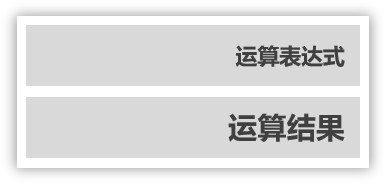``````@Component
export struct InPutComponent {
private isLand: boolean
build() {
Stack({ alignContent: this.isLand ? Alignment.BottomStart : Alignment.TopEnd }) {
Column() {
//运算表达式文本框
Scroll() {
Text(this.expression)
.maxLines(1)
.opacity(0.9)
.fontWeight(400)
.textAlign(TextAlign.Start)
.fontSize(this.isLand ? 50 : 35)
}
.width('90%')
.scrollable(ScrollDirection.Horizontal)
.align(this.isLand ? Alignment.Start : Alignment.End)
//运算结果文本框
Scroll() {
Text(this.result)
.maxLines(1)
.opacity(0.38)
.textAlign(TextAlign.Start)
.fontSize(this.isLand ? 45 : 30)
.margin(this.isLand ? { bottom: 64 } : {})
}
}
}
}
}``````

1. 菜单栏模块

“菜单栏”模块为计算器顶部菜单栏，是计算器分布式功能的入口。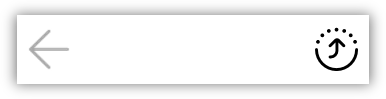``````@Component
export struct TitleBar {
build() {
Row() {
Image(\$r("app.media.ic_back"))
.height('60%')
.margin({ left: 32 })
.width(this.isLand ? '5%' : '8%')
.objectFit(ImageFit.Contain)
//执行terminate()方法，退出计算器应用
.onClick(() => {
app.terminate()
})
Blank().layoutWeight(1)
Image(\$r("app.media.ic_hop"))
.height('60%')
.margin({ right: 32 })
.width(this.isLand ? '5%' : '8%')
.objectFit(ImageFit.Contain)
//执行showDialog()方法，界面上弹出的分布式设备列表弹窗
.onClick(() => {
this.showDiainfo()
})
}
.width('100%')
.height(this.isLand ? '10%' : '8%')
.constraintSize({ minHeight: 50 })
.alignItems(VerticalAlign.Center)
}
}``````

2. 分布式设备管理

(1) 分布式设备搜索

``````startDeviceDiscovery() {
SUBSCRIBE_ID = Math.floor(65536 * Math.random())
let info = {
subscribeId: SUBSCRIBE_ID,
mode: 0xAA,
medium: 2,
freq: 2,
isSameAccount: false,
isWakeRemote: true,
capability: 0
}
Logger.info(TAG, `startDeviceDiscovery \${SUBSCRIBE_ID}`)
this.deviceManager.startDeviceDiscovery(info)
}``````

(2) 分布式设备列表弹窗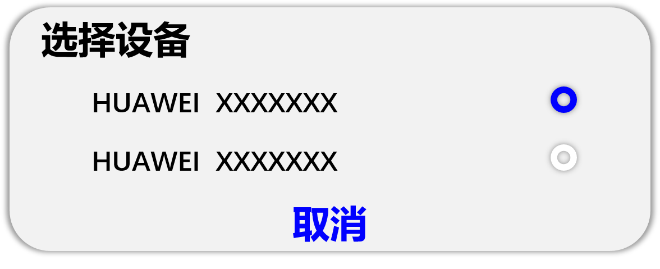``````@CustomDialog
export struct DeviceDialog {
build() {
Column() {
List() {
ForEach(this.deviceList, (item, index) => {
ListItem() {
Row() {
Text(item.deviceName)
.fontSize(21)
.width('90%')
.fontColor(Color.Black)
Image(index === this.selectedIndex ? \$r('app.media.checked') : \$r('app.media.uncheck'))
.width('8%')
.objectFit(ImageFit.Contain)
}
.height(55)
.margin({ top: 17 })
.onClick(() => {
if (index === this.selectedIndex) {
return
}
this.selectedIndex = index
this.onSelectedIndexChange(this.selectedIndex)
})
}
}, item => item.deviceName)
}
}
}
}``````

(3) 远端设备拉起

``````startAbility(deviceId) {
featureAbility.startAbility({
want: {
bundleName: 'ohos.samples.DistributeCalc',
abilityName: 'ohos.samples.DistributeCalc.MainAbility',
deviceId: deviceId,
parameters: {
isFA: 'FA'
}
}
}).then((data) => {
this.startAbilityCallBack(DATA_CHANGE)
})
}``````

3. 分布式数据管理

(1) 管理分布式数据库

``````async createKvStore(callback) {
//创建一个KVManager对象实例
this.kvManager = await distributedData.createKVManager(config)
let options = {
createIfMissing: true,
encrypt: false,
backup: false,
autoSync: true,
kvStoreType: 1,
securityLevel: 1,
}
// 通过指定Options和storeId，创建并获取KVStore数据库，并通过Promise方式返回，此方法为异步方法。
this.kvStore = await this.kvManager.getKVStore(STORE_ID, options)
callback()
}``````

(2) 订阅分布式数据变化

``````kvStoreModel.setOnMessageReceivedListener(DATA_CHANGE, (value) => {
if (this.isDistributed) {
if (value.search(EXIT) != -1) {
Logger.info(TAG, `EXIT \${EXIT}`)
featureAbility.terminateSelf((error) => {
Logger.error(TAG, `terminateSelf finished, error= \${error}`)
});
} else {
this.expression = value
if (this.isOperator(this.expression.substr(this.expression.length - 1, this.expression.length))) {
this.result = JSON.stringify(MATH.evaluate(this.expression.substr(0, this.expression.length - 1)))
} else {
this.result = JSON.stringify(MATH.evaluate(this.expression))
}
}
}
})``````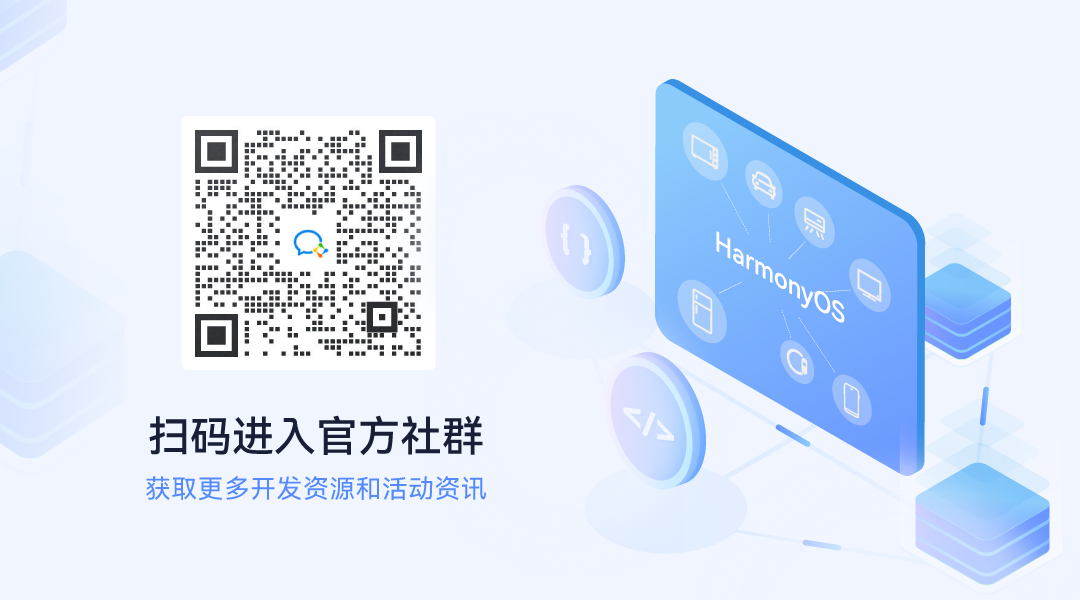2

1条回复

/

wx621885b50f4da

2022-5-28 10:16:20帖子
视频
声望
粉丝
最近发布
相关问题
社区精华内容